JEE  >  Test: Binomial Theorem For Positive Index

# Test: Binomial Theorem For Positive Index

Test Description

## 15 Questions MCQ Test Mathematics (Maths) Class 11 | Test: Binomial Theorem For Positive Index

Test: Binomial Theorem For Positive Index for JEE 2023 is part of Mathematics (Maths) Class 11 preparation. The Test: Binomial Theorem For Positive Index questions and answers have been prepared according to the JEE exam syllabus.The Test: Binomial Theorem For Positive Index MCQs are made for JEE 2023 Exam. Find important definitions, questions, notes, meanings, examples, exercises, MCQs and online tests for Test: Binomial Theorem For Positive Index below.
Solutions of Test: Binomial Theorem For Positive Index questions in English are available as part of our Mathematics (Maths) Class 11 for JEE & Test: Binomial Theorem For Positive Index solutions in Hindi for Mathematics (Maths) Class 11 course. Download more important topics, notes, lectures and mock test series for JEE Exam by signing up for free. Attempt Test: Binomial Theorem For Positive Index | 15 questions in 15 minutes | Mock test for JEE preparation | Free important questions MCQ to study Mathematics (Maths) Class 11 for JEE Exam | Download free PDF with solutions
 1 Crore+ students have signed up on EduRev. Have you?
Test: Binomial Theorem For Positive Index - Question 1

### The number of terms in the expansion of (2x - 3y)8 is

Detailed Solution for Test: Binomial Theorem For Positive Index - Question 1

Since this binomial is to the power 8, there will be nine terms in the expansion.

Test: Binomial Theorem For Positive Index - Question 2

### The middle term in the expansion of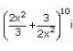Detailed Solution for Test: Binomial Theorem For Positive Index - Question 2

n = 10

Middle term = (n/2) + 1
= (10/2) + 1
= 6th term

T(6) = T(5+1)
= 10C5[(2x2)/3]5 [(3/2x2)]5
= 10C5
= 252

Test: Binomial Theorem For Positive Index - Question 3

### In the expansion of (a+b)n, N the number of terms is:

Detailed Solution for Test: Binomial Theorem For Positive Index - Question 3

The total number of terms in the binomial expansion of (a + b)n is n + 1, i.e. one more than the exponent n.

Test: Binomial Theorem For Positive Index - Question 4

Find the value of r, if the coefficients of (2r + 4)th and (r – 2)th terms in the expansion of (1 + x)18 are equal.

Detailed Solution for Test: Binomial Theorem For Positive Index - Question 4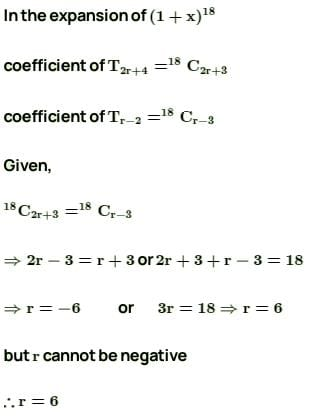Test: Binomial Theorem For Positive Index - Question 5

The sixth term in the expansion of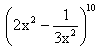is

Detailed Solution for Test: Binomial Theorem For Positive Index - Question 5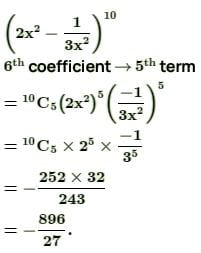Test: Binomial Theorem For Positive Index - Question 6

If the coefficients of 7th and 13th terms in the expansion of (1 + x)n are equal, then n is equal to

Detailed Solution for Test: Binomial Theorem For Positive Index - Question 6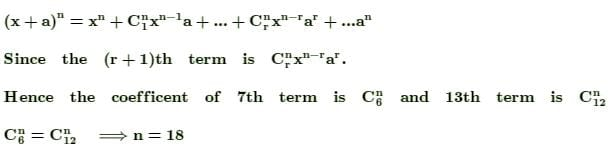Test: Binomial Theorem For Positive Index - Question 7

The 6th term in the expansion of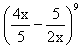is

Detailed Solution for Test: Binomial Theorem For Positive Index - Question 7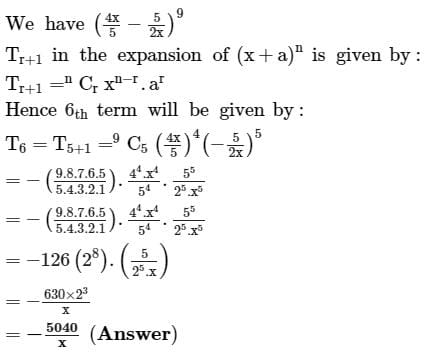Test: Binomial Theorem For Positive Index - Question 8

What is the coefficient of x5 in the expansion of (1-x)-6 ?

Detailed Solution for Test: Binomial Theorem For Positive Index - Question 8

(1-x)-6
=> (1-x)(-6/1)
It is in the form of (1-x)(-p/q), p =6, q=1

(1-x)(-p/q) = 1+p/1!(x/q)1 + p(p+q)/2!(x/q)2 + p(p+q)(p+2q)/3!(x/q)3 + p(p+q)(p+2q)(p+3q)/4!(x/q)4........

= 1+6/1!(x/1)1 + 6(7)/2!(x/1)2 + 6(7)(8)/3!(x/1)3 + 6(7)(8)(9)/4!(x/1)4 +.......................

So, coefficient of x5 is (6*7*8*9*10)/120
= 252

Test: Binomial Theorem For Positive Index - Question 9

In the expansion of the binomial expansion (a + b)n, which of the following is incorrect ?

Detailed Solution for Test: Binomial Theorem For Positive Index - Question 9

Explanation:- The coefficient of terms (x+a)n equidistant from the beginning and the end are equal. These coefficients are known as the binomial coefficients.

nCr = nCn – r, r = 0,1,2,…,n.

Test: Binomial Theorem For Positive Index - Question 10

The middle term in the expansion of (x + y)10 is the

Detailed Solution for Test: Binomial Theorem For Positive Index - Question 10

Number of terms(n) = 10
Middle term = (n/2) + 1

= (10/2) + 1
= 5 + 1
= 6th term

Test: Binomial Theorem For Positive Index - Question 11

In a binomial expansion with power 13

Detailed Solution for Test: Binomial Theorem For Positive Index - Question 11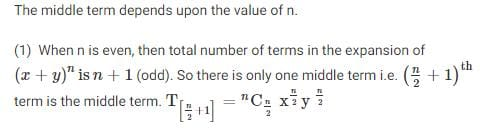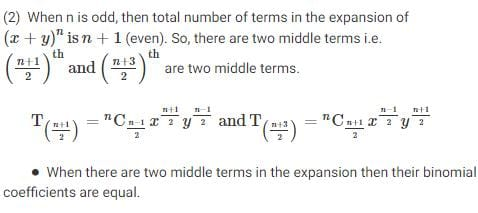Test: Binomial Theorem For Positive Index - Question 12

If in the expansion of (1+x)20, the coefficients of rth and (r+4)th terms are equal, then the value of r is equal to:

Detailed Solution for Test: Binomial Theorem For Positive Index - Question 12

Coefficients of the rth and (r+4)th terms in the given expansion are Cr−120  and 20Cr+3.
Here,Cr−120  = 20Cr+3
⇒ r−1+r+3 = 20
[∵ if nCnCy  ⇒ x = y or x+y = n]

⇒ r = 2 or 2r = 18
⇒ r = 9

Test: Binomial Theorem For Positive Index - Question 13

The number of terms in the expansion of (x – y + 2z)7 are:

Detailed Solution for Test: Binomial Theorem For Positive Index - Question 13

Here the number of terms can be calculated by:
= ((n+ 1) * (n+2)) /2
where, n =7

∴ Number of terms = 36

Test: Binomial Theorem For Positive Index - Question 14

The number of terms in the expansion of (a + b + c)n are:

Detailed Solution for Test: Binomial Theorem For Positive Index - Question 14

No. of terms is n+2C2

Test: Binomial Theorem For Positive Index - Question 15

The general term in the expansion of (a - b)n is

Detailed Solution for Test: Binomial Theorem For Positive Index - Question 15

If a and b are real numbers and n is a positive integer, then:
(a - b)n = nC0 an + nC1 a(n – 1) b1 + nC2 a(n – 2) b2+ ...... + nCr a(n – r) br+ ... + nCnbn,

The general term or (r + 1)th term in the expansion is given by:
Tr + 1 = (-1)Cr a(n–r) br

## Mathematics (Maths) Class 11

156 videos|176 docs|132 tests
 Use Code STAYHOME200 and get INR 200 additional OFF Use Coupon Code
Information about Test: Binomial Theorem For Positive Index Page
In this test you can find the Exam questions for Test: Binomial Theorem For Positive Index solved & explained in the simplest way possible. Besides giving Questions and answers for Test: Binomial Theorem For Positive Index, EduRev gives you an ample number of Online tests for practice

## Mathematics (Maths) Class 11

156 videos|176 docs|132 tests